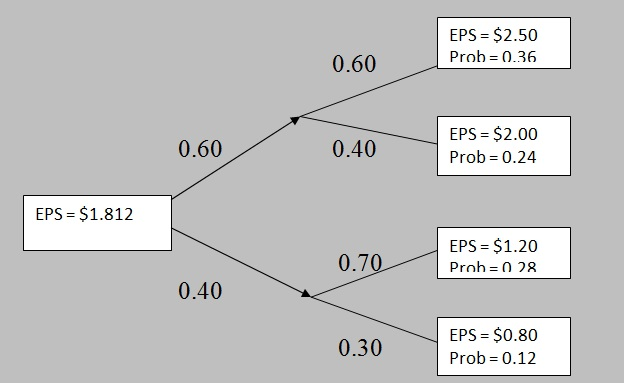# Tree diagram representing an investment problem

CFA level I / Quantitative Methods: Basic Concepts / Probability Concepts / Tree diagram representing an investment problem

The tree diagram makes it very easy to calculate the expected value of a random variable.

 Example 4: Calculating expected value using Tree diagram The EPS of a company is dependent on the statement of the economy. If the economy is good, then there is 60 percent probability that the EPS will be \$2.5 and 40 percent probability that the EPS will be \$2.0. If the economy is bad, then there is 70 percent probability that the EPS will be \$1.2 and 30 percent probability that the EPS will be \$0.8. The probability of occurrence of a good economy is 60 percent, and that of a bad economy is 40 percent. Calculate the expected value of the company's EPS using a tree diagram. Solution:The conditional value of EPS when the economy is good = 0.60*\$2.5 + 0.40*\$2.0 = \$2.30 The conditional value of EPS when the economy is bad = 0.70*\$1.2 + 0.30*\$0.8 = \$1.08 The unconditional value of EPS = 0.60*\$2.30 + 0.40*\$1.08 = \$1.812 Using tree diagram: Probability of EPS to be equal to \$2.50 = 0.60*0.60 = 0.36 (upper-upper node) Probability of EPS to be equal to \$2.00 = 0.60*0.40 = 0.24 (upper-lower node) Probability of EPS to be equal to \$1.20 = 0.40*0.70 = 0.28 (lower-upper node) Probability of EPS to be equal to \$0.80 = 0.40*0.30 = 0.12 (lower-lower node) Please note that the sum of probabilities of all nodes is equal to one. Expected value of EPS = 0.36*\$2.50 + 0.24*\$2.00 + 0.28*\$1.20 + 0.12*\$0.80 = \$1.812

CFA Institute does not endorse, promote or warrant the accuracy or quality of products and services offered by Konvexity. CFA® and Chartered Financial Analyst® are registered trademarks owned by CFA Institute.